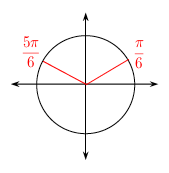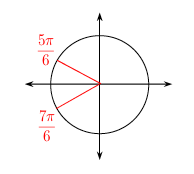### Home > A2C > Chapter 13 > Lesson 13.1.2 > Problem13-31

13-31.

For each of the following equations, find the solutions that lie in the domain $0 ≤ x < 2π$.

1. $2\sin(x) - 1 = 0$

For the equation to be true, $\sin(x)$ must $=\frac{1}{2}$.

You can either draw a unit circle to find all possible solutions or solve the equation graphically.2. $2\cos(x) = -\sqrt { 3 }$

In what quadrants is cos$(x)$ negative?3. $2\sin(x) =\sqrt { 2 }$

When does $\sin(x)=\frac{\sqrt{2}}{2}$?

4. $\cos(x) = 1$

Picture a unit circle. At what angle is the adjacent side the same length as the hypotenuse?